Full Text:<1607>

Summary:<1243>

CLC number: O344

On-line Access: 2014-07-08

Revision Accepted: 2014-05-08

Crosschecked: 2014-06-24

Cited: 4

Clicked: 7957

Citations:  Bibtex RefMan EndNote GB/T7714

 Journal of Zhejiang University SCIENCE A 2014 Vol.15 No.7 P.482-495 http://doi.org/10.1631/jzus.A1400031Influence of actual plastic hinge placement on the behavior of ductile frames

 Author(s):  Massimiliano Fraldi, Antonio Gesualdo, Federico Guarracino Affiliation(s):  . Department of Structural Engineering, University of Naples Federico II, 80125, Napoli, Italy Corresponding email(s):   fguarrac@unina.it Key Words:  Steel frames, Limit analysis, Actual plastic hinge localisation, Collapse kinematics Share this article to： More <<< Previous Article|Next Article >>>

Massimiliano Fraldi, Antonio Gesualdo, Federico Guarracino. Influence of actual plastic hinge placement on the behavior of ductile frames[J]. Journal of Zhejiang University Science A, 2014, 15(7): 482-495.

@article{title="Influence of actual plastic hinge placement on the behavior of ductile frames",
author="Massimiliano Fraldi, Antonio Gesualdo, Federico Guarracino",
journal="Journal of Zhejiang University Science A",
volume="15",
number="7",
pages="482-495",
year="2014",
publisher="Zhejiang University Press & Springer",
doi="10.1631/jzus.A1400031"
}

%0 Journal Article
%T Influence of actual plastic hinge placement on the behavior of ductile frames
%A Massimiliano Fraldi
%A Antonio Gesualdo
%A Federico Guarracino
%J Journal of Zhejiang University SCIENCE A
%V 15
%N 7
%P 482-495
%@ 1673-565X
%D 2014
%I Zhejiang University Press & Springer
%DOI 10.1631/jzus.A1400031

TY - JOUR
T1 - Influence of actual plastic hinge placement on the behavior of ductile frames
A1 - Massimiliano Fraldi
A1 - Antonio Gesualdo
A1 - Federico Guarracino
J0 - Journal of Zhejiang University Science A
VL - 15
IS - 7
SP - 482
EP - 495
%@ 1673-565X
Y1 - 2014
PB - Zhejiang University Press & Springer
ER -
DOI - 10.1631/jzus.A1400031

Abstract:
The ultimate load and collapsing modes of steel frames under combined vertical and horizontal forces are investigated through finite element (FE) modelling and limit analysis. Consideration is given to a frequently overlooked problem which is the kinematics arising from the actual rotation of the plastic hinges under axial force and bending moment. This fact draws attention to the necessity of a careful assessment of the limit analysis approaches, a fact that might be seen as somewhat in line with the outcome from famous paradoxes, such as the one by Stüssi and Kollbrunner (1935), which can only be solved by making reference to both elastic and plastic deformations. As a result, it can be shown that in such a manner, it is possible to obtain a handy tool capable of competing with much more computationally expensive methodologies. The approach may be relevant to practising engineers dealing with code prescriptions and standardization committees.

1.  Introduction

The collapse factor represents an important outcome of a plastic structural analysis and it is a significant parameter in the framework of the safety assessment and economical design of ductile structures.

From this point of view, classic limit analysis is concerned with the problem of finding how resistant a given structure is with the aim to estimate the factor by which the loads component needs to be amplified so that a structural crisis occurs, which takes the form of plastic collapse (Chen, ). A plastic collapse takes place when the structure is converted into a mechanism by the development of a suitable number and disposition of plastic hinges. To apply the methods of limit analysis, however, a very simplified and idealised model of the structure must be adopted and, notwithstanding the fact that hundreds of papers have been devoted to the topic, some consequences of apparently unimportant simplifications still seem to have not been properly and firmly highlighted. To get a perspective of the research made in this field and of the main issues concerning the real elastic-plastic behaviour of steel structures and of the influence of simplifying hypotheses adopted in the limit analysis methods, reference can be made to Petrolito and Legge (). They present a review of previous works related to different methods for plastic analysis of steel frames, including “various approximate theories that can be derived from the general non-linear theory” and “high-accuracy solutions to a series of benchmark problems for a variety of modelling assumptions”. Several different approaches for different types of plastic analysis are presented, discussed or referenced in some books and reports (Massonnet and Save, ; Halleux, ; Save et al., ). The effects of strain hardening, local buckling, limit deformations, position of the neutral axis and bending moment-axial force interaction on the formation and position of plastic hinges, or on the spreading of the yielded zones are covered (Davies, ; ; Baptista and Muzeau, ; ; Abbas and Jones, ).

Possible shortcomings of limit analysis clearly emerge from well-known paradoxes, such as the one by Stüssi and Kollbrunner (), which can generally be solved only by making reference to a methodology comprising both elastic and plastic deformations.

On the other hand, the collapse factor can also be computed in a step-by-step fashion by following the evolution of the inelastic structural response of a suitably discretized structure to a given loading history (Chen, ), but such time-stepping analyses are computationally demanding and unsuitable for design or assessment purposes in the normal engineering practice. Additionally, they need accurate modelling and interpretation of results. For this reason, many codes for the design of steel building frames, such as Eurocode 3 (EC3, ; Gardner and Nethercot, ; Trahair et al., ) make references to limit analysis for design and assessment of the capacity loading of steel structures under combined static and seismic loadings.

In the present study it is shown, by means of some simple examples, that, if references are not made to the actual kinematics arising from the plastic hinges on account of the axial force-bending moment interaction, the safety factors of the frame structure may be significantly affected, and also in common loading cases. More importantly, different behaviours of the plastic hinges may give origin to different collapse mechanisms, and thus render this primary information from limit analysis misleading for the design of new structures and for the strengthening of existing ones.

To this purpose, both the commercially available finite element (FE) package ANSYS® (ANSYS, ) and a purposely written automatic implementation of the limit analysis procedures (Cohn and Maier, ) within the framework of the symbolic code MATHEMATICA® (Wolfram, ) have been employed. The ultimate loading capacity of a few structures has been analysed and discussed. The methodology is presented in the form of a handy tool which can be acceptable to practising engineers and help them to analyse the effects of the design parameters of the cross section on the ultimate load and the actual kinematics at collapse in a direct and very effective way. This is done in much the same spirit of a simplified analysis of the influence of soil-structure interaction proposed in the past by one of the present authors (Guarracino et al., ).

Through advancing classical procedures and focusing on a specific aspects, this paper highlights the need for engineers dealing with limit analysis within the framework of current European codes to be aware of the possibility that a local approximation in a very idealised model of a structure may unexpectedly give origin to different collapse kinematics, a fact which is acknowledged in stability analyses for a broad class of structural members (Guarracino and Walker, ). The intention is also to add practical knowledge to some recent theoretical investigations (Fraldi et al., ).

2.  Methods of plastic analysis of ductile frames

Broadly speaking, engineers have two categories of methods at their disposal for the assessment of collapse analysis of ductile frames: the finite element nonlinear step-by-step methods and the limit analysis methods of plasticity. The FE analyses are more general, but can be very time-consuming, depending on the degree of discretization applied. In fact, dividing beams and columns into a fine mesh of elements may give indications about the actual localization of plasticity and failure but also become rapidly unfeasible from a computational standpoint for the overall analysis of multi-story buildings. Also, convergence and stability issues in very large problems may also lead to incorrect results. On the contrary, limit analysis methods are focused on the determination of the global safety margins of the structure and lead to the solution of much simpler convex optimization problems.

Limit analysis is based on the rigid-plastic theory in which the plastic collapse load is evaluated through a virtual work formulation and elastic deflection is ignored and, although there has been restrictions in its use, it has merits for the ultimate assessment of ductile frames, especially in the case of extreme scenarios, propped by unforeseen events which are impossible to be incorporated in a computational model via usual loading concepts, given that any data for them are absent and the associated uncertainties are significant. An inverse way of simulation is therefore recommended by most guidelines and can often be effectively pursued by limit analysis methods, as it is the case, for example, of the so-called column loss, which suggests the analysis of the structural system incorporating the absence of an important part of the structure, like a column, implying that the specific element is completely damaged as a result of an abnormal event (Kim and Huh, ; Kwasniewski, ).

However, as anticipated in Section 1, all the simplifications at the basis of the procedures might be sometimes overlooked and not properly accounted in a much idealised modeling of the structure and as such they require careful attention. This is the case of the effects of the plastic hinges localization through the cross section on the collapse load and kinematics of the structure.

To this purpose, in what follows, two elementary examples will be first discussed and a comparison will also be drawn between the results from a non-linear FE analysis and the limit analysis procedure. Successively, the focus will be set on a particular automation of the static method by means of the symbolic code MATHEMATICA® which will show how it is possible to derive the collapse kinematics on account of actual positioning of the plastic hinges through the cross section in a very simple and direct manner.

3.  Design of steel structures according to EC3 and axial force-bending moment interaction

The cross section of frame columns is usually biaxial symmetric because frame columns are often subjected to biaxial bending (Fig. 1).

Over the years several criteria for the analysis of steel I-sections subjected to axial forces and bending moments have been proposed (Baptista, ), but for the present purposes the initial yielding surface of a column section can be determined by linear superimposition of normal stresses within the elastic scope and can be written as follows: , where Mx and My are the bending moments applied about the x-axis and y-axis, respectively, N is the axial force applied, and Mx o, My o, and N o are the full yielded cross-section resistances when Mx , My , and N are applied alone, respectively. For simplicity, Fig. 2 shows the linearized yield domain when only one bending moment, either about the x-axis or the y-axis, is applied. It is worth noticing that the yield domain of Fig. 2 corresponds to a full yielded cross-section resistance criterion and is in actual fact a linearization of an intrinsically non-linear interaction curve. As a matter of fact, this linearized approach can be generally regarded as conservative even if the additional resistance reserve, up to the real non-linear criterion, is larger in the case of a cross-section subject to a small axial force and a considerable bending moment, than in the case of a cross-section subject to a large axial force and a small bending moment. Additionally, the formation of the plastic hinge is more gradual in the first case than in the second one. As a consequence, this simplifying hypothesis may render the cross-section bending mechanizms more frequent in the limit analysis than they really are in practice. However, the yield domain of Fig. 2 is customarily employed in limit analyses (Massonnet and Save, ; Chen, ) and its adoption allows a substantial simplification of all the calculations. Given that such a simplification has been proven reliable in a large number of real-world cases and is normally prescribed by several codes, the present treatment will be based on its implementation with the explicit warning that all the results, as it is normally the case in limit analysis, must be carefully judged and assessed in the factual practice.Fig.2
Idealized yield domain in the M-N plane for an I section

It is worth noting that the Eurocode 3 (EC3, ) requires that the plastic hinges must ensure the real capacity to develop the necessary rotations, but it does not explicitly make reference to the M-N interaction with respect to limit mechanisms.

In fact, the EC3 () states that the global plastic analysis can be performed by means of rigid-plastic or elastic-plastic analyses and that the plastic hinge can occur in the column or beam with sufficient rotation capacity.

Conversely, EC3 explicitly deals with M-N interaction with reference to transverse sections of the structural elements of classes 1 and 2 and with reference to the interaction M-N-T, albeit without any kinematic consideration.

Overall, it is important to realize that this fact may lead to an incorrect evaluation of the problem, especially if the collapsing behavior is drawn from the elastic solution, as shown in the elementary examples which follow.

3.1.  Example 1: axial force-bending moment interaction in a statically determined horizontal beam

The collapse factor for a simply supported beam considered under pure bending (that is, neglecting the effects of the axial force on the ultimate resistance of the cross section) (Fig. 3), can be straightforwardly obtained by means of the static theorem of limit analysis (Neal, ) by prolonging the vector representing the M-N status at the section B in the elastic range until the horizontal line through M o is met, as shown in Fig. 4.Fig.3
Example 1: a simple supported beam
α is the axial force multiplier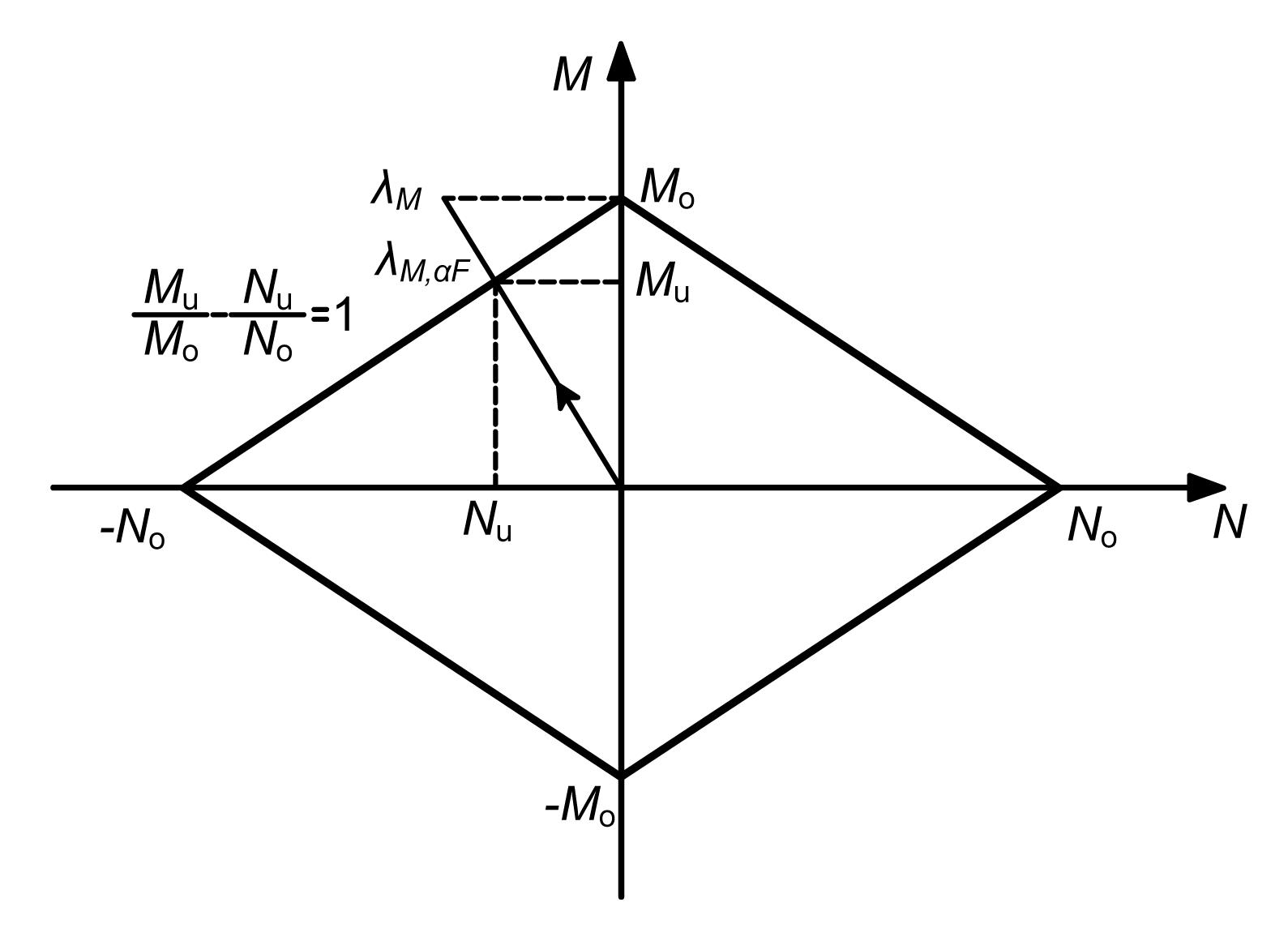Fig.4
Example 1: yield condition at a section for pure bending or combined bending and axial forces
N u and M u are the values of N and M on the yielding locus

Thus, it is .

Conversely, the collapse load multiplier for the same simply supported beam but accounting for both bending and axial forces is obtained in the same fashion by prolonging the vector representing the M-N status at the section B in the elastic range until the yield locus is met (Fig. 4).

It follows .

The same results are obtained by means of the kinematic theorem (Neal, ) (Fig. 5). In fact, under pure bending, the result Eq. (2) is given again by the virtual work equation. It is worth noticing that in such a case the rotation at the plastic hinges must be assumed to take place with the pins at the center of the mass of the cross section, as shown in Fig. 5 (C 1, C 12, C 2).Fig.5
Example 1: kinematics at collapse for pure bending

In the same fashion, the influence of the axial force, , can be taken into account by considering that the rotations at the plastic hinges must take place about the pins at the neutral axis of the fully plastic cross section at section B (Fig. 6).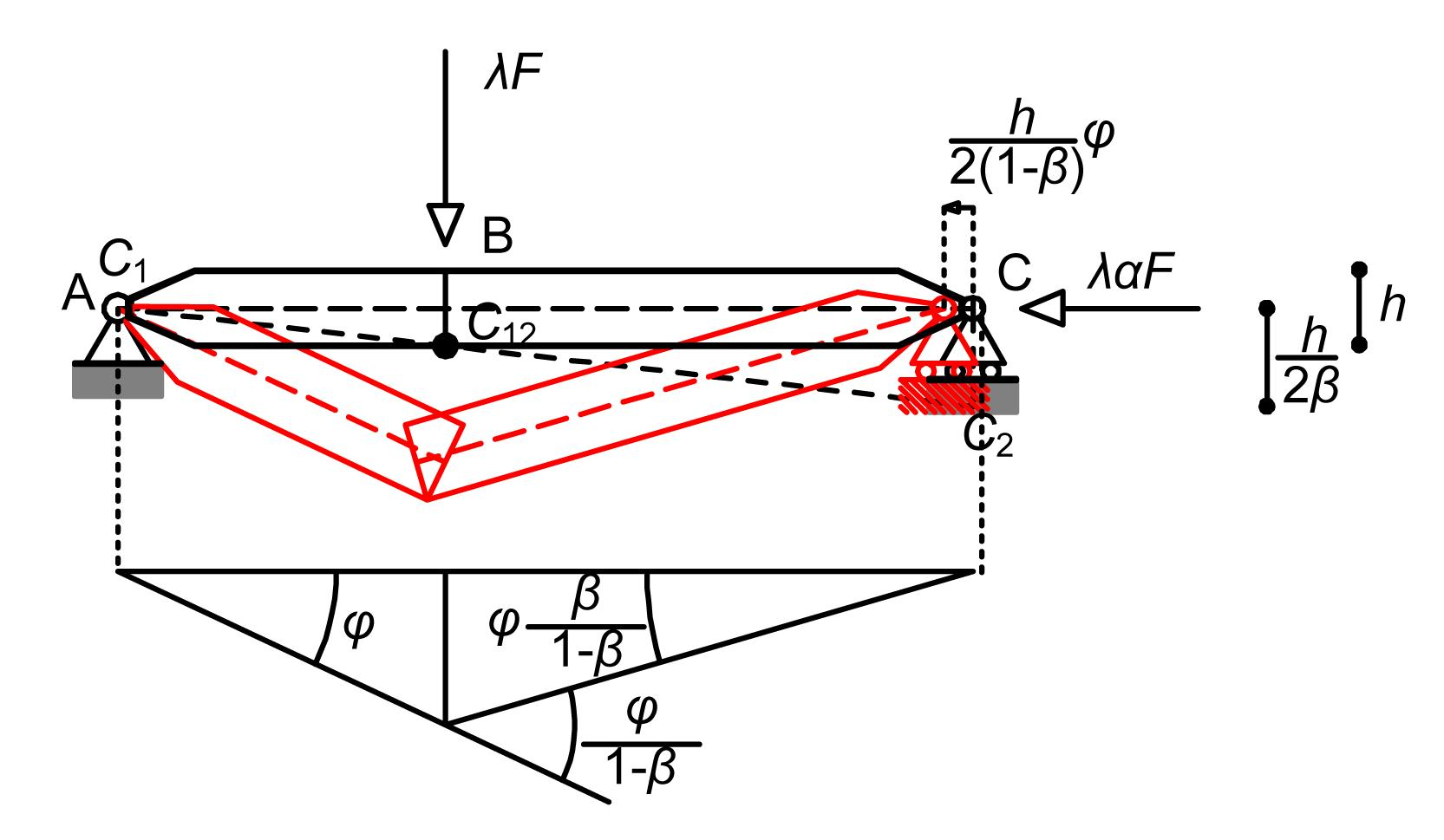Fig.6
Example 1: kinematics at collapse for bending moment and axial force interaction
h is the height of the cross section

Therefore, the virtual work equation yields Eq. (3) again.

3.2.  Example 2: axial force-bending moment interaction in a statically undetermined horizontal beam

Different from the case of a statically determined system, for which the statically admissible stress status is unique and derives from the solution of the equilibrium equations, in the case of a statically undetermined system, as the supported cantilever of Fig. 7, the collapse factor under pure bending cannot be obtained by means of the static theorem by prolonging the vectors representing the M-N elastic status at the sections A and B until the horizontal line through M o is met, as shown in Figs. 8a and 8b.Fig.8
Example 2: different yield conditions at sections A and B.
(a) and (b): pure bending; (c) and (d): bending and axial force interaction

In fact, in such a case, either the prolongation of the vector representing the elastic solution at section A (for ) or at B (for ) will first meet the horizontal line for ±M o. At this point, the structure changes configuration on account of the presence of a plastic hinge at section A or B, respectively, and the attainment of the collapsing status takes place following a different path, as shown in Figs. 8a and 8b. It is .

The same line of reasoning can be applied when both bending and axial forces are taken into account for determining the yield status of the cross section and the situation is shown in Figs. 8c and 8d. The collapse factor results are different from the one obtained by prolonging the vector representing the M-N status at the sections A and B in the elastic range until the yield locus is met.

In fact, it is given in Eq. (5) that , where we can obtain from Eq. (7) that .

The results Eqs. (4) and (6) can be straightforwardly obtained though the kinematic theorem and the virtual work equation with references, as shown in Figs. 9 and 10 and yet again it is clear that the rotations at the plastic hinges must take place about the pins at the neutral axis of the fully plastic cross section.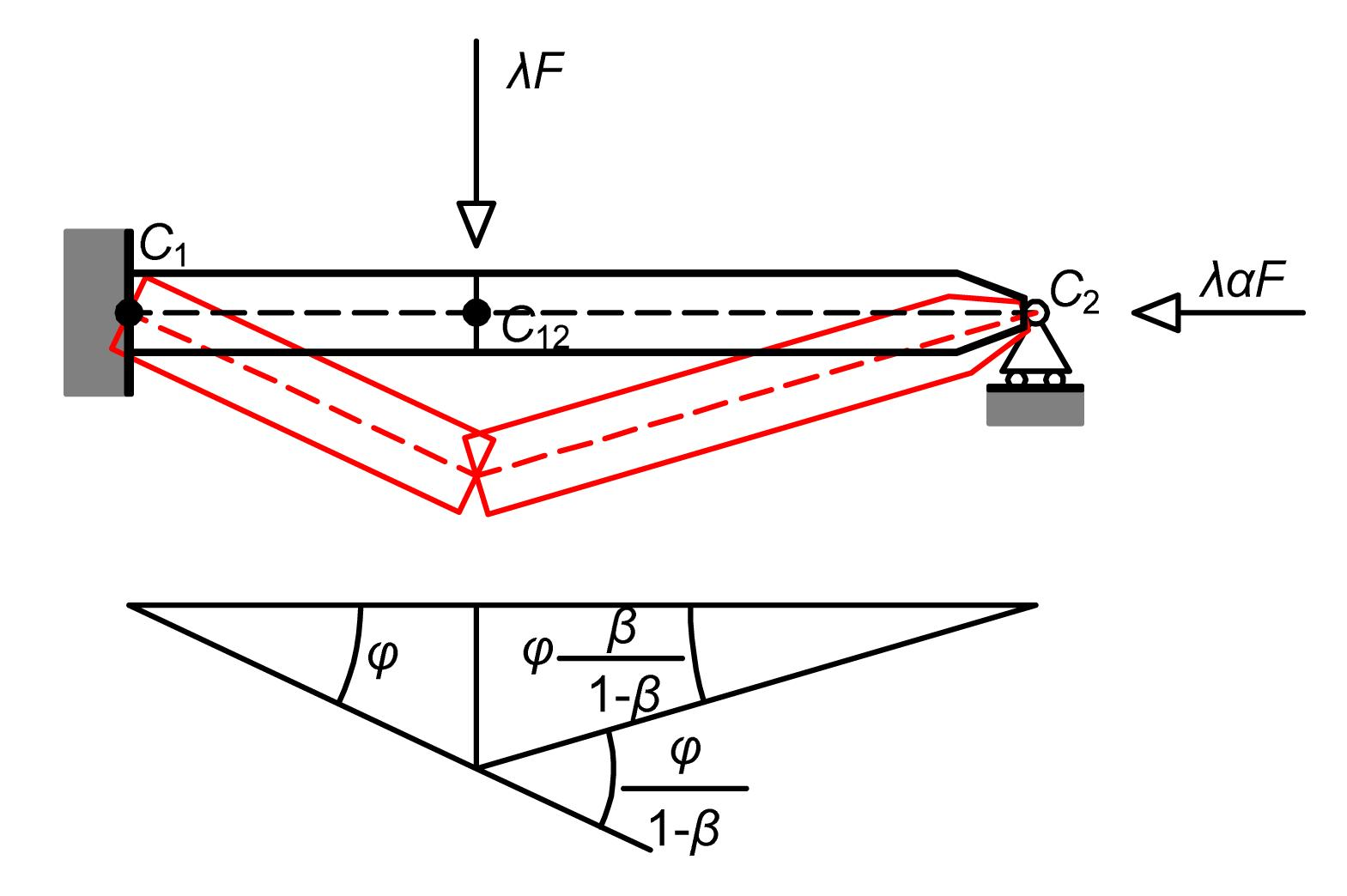Fig.9
Example 2: kinematics at collapse for pure bending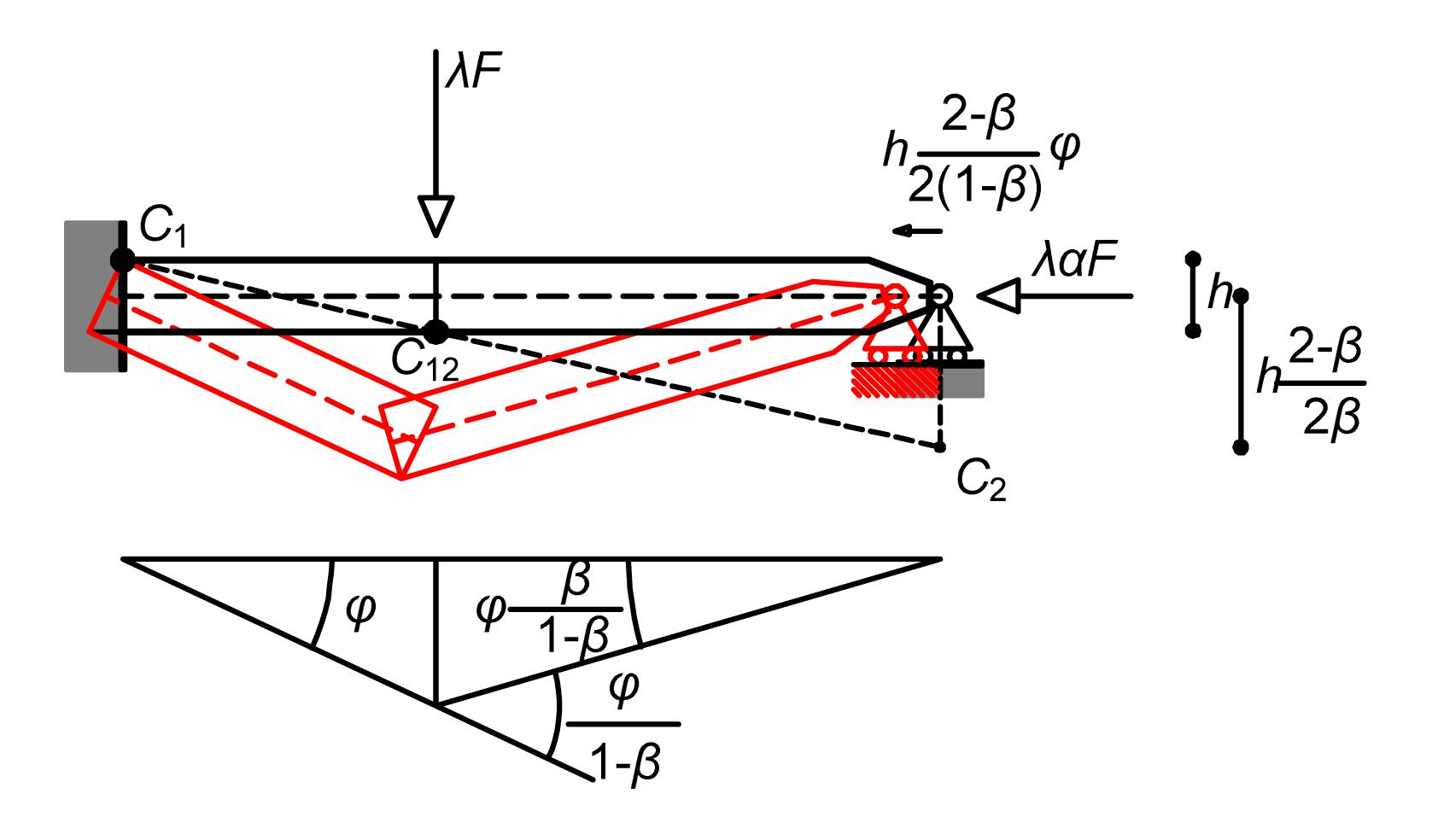Fig.10
Example 2: kinematics at collapse for bending moment and axial force interaction

It is worth mentioning that the difference between the limit load multipliers Eqs. (5) and (6) varies with the position of the force F, as shown in Fig. 11 for the specified parameters.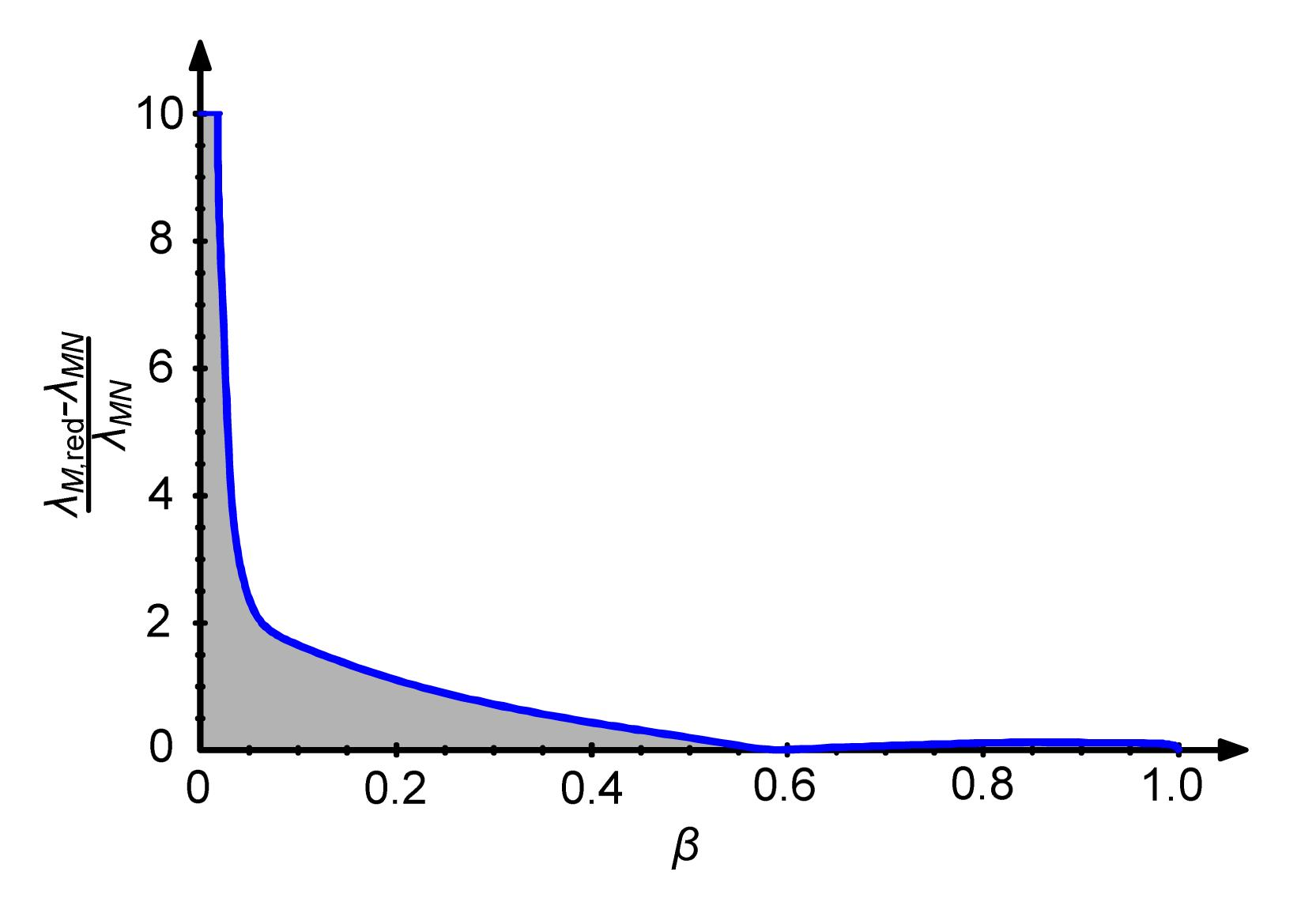Fig.11
Variation of multipliers and for the supported cantilever of Fig. 7 according to the position of the point load
(Section: HE160A, σ o=440 MPa, M o=150.34 kN·m, N o=2302.08 kN, F o=150 kN, L=5 m, and α=0.2)

4.  An assessment of kinematics at collapse through nonlinear finite elements

To assess the results from a highly idealized limit analysis of the statically undetermined frame of Fig. 12 by means of a refined nonlinear FE analysis and to discuss its kinematics at collapse, the commercially available finite element package ANSYS® has been employed.Fig.12
A simple portal frame
(Section HE140A, σ o=355 MPa, M o=59.16 kN·m, N o=1071.4 kN, L=5 m, H 1=3.5 m, H 2=1.75 m, q=10 kN/m, F o=150 kN, F v=7 kN, β=0.3, and γ=0.25)

The mesh and the loading of the structure are shown in Figs. 13 and 14, respectively. The simple portal has been modeled by means of 42 000 SOLID 185 elements. SOLID 185 is defined by eight nodes with three degrees of freedom (DOFs) at each node: translations in the nodal x, y, and z directions. The element has plasticity, large deflection, and large strain capabilities (ANSYS, ).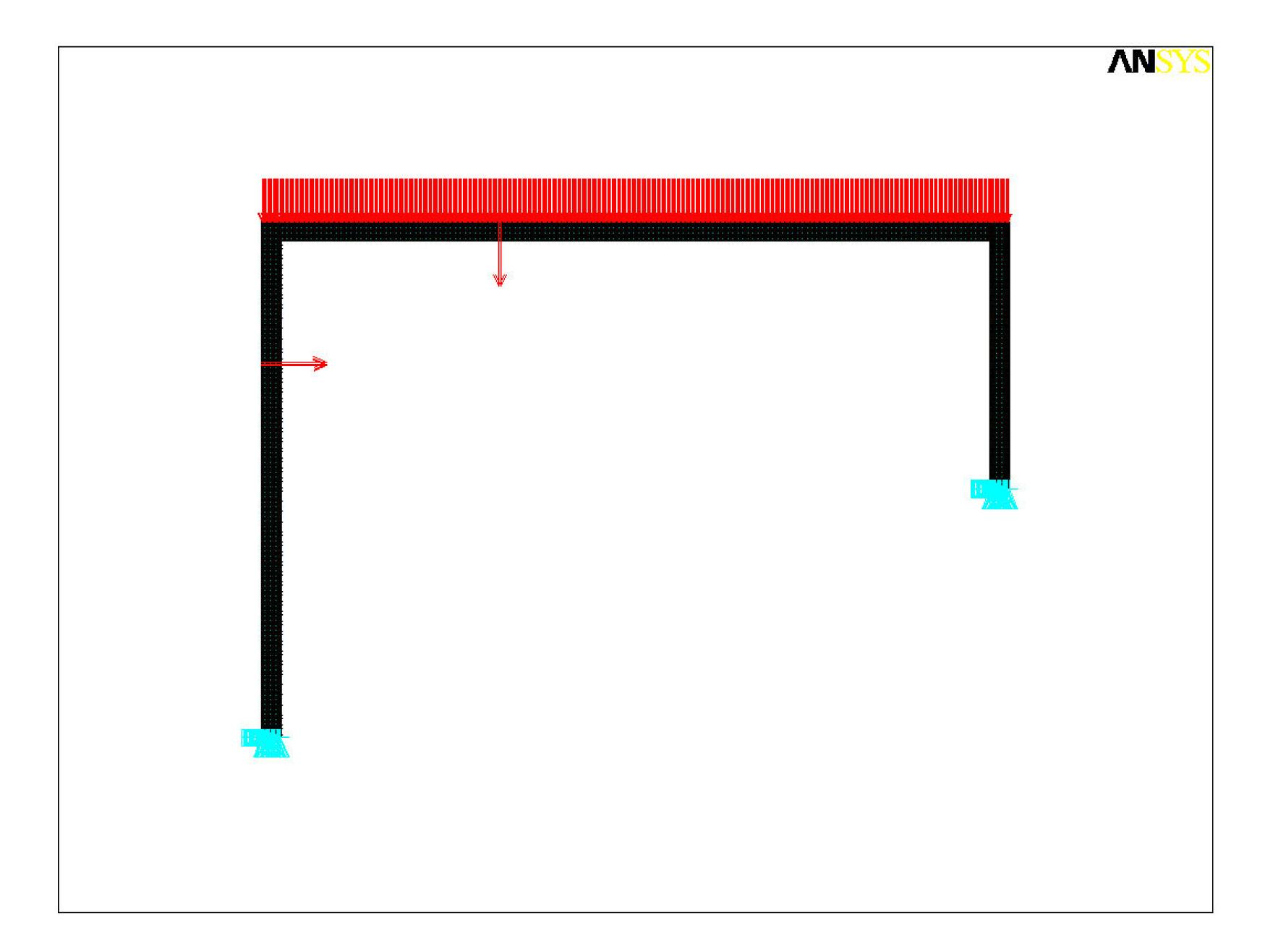Fig.13
Finite element model of the simple portal of Fig. 12: loading and constraints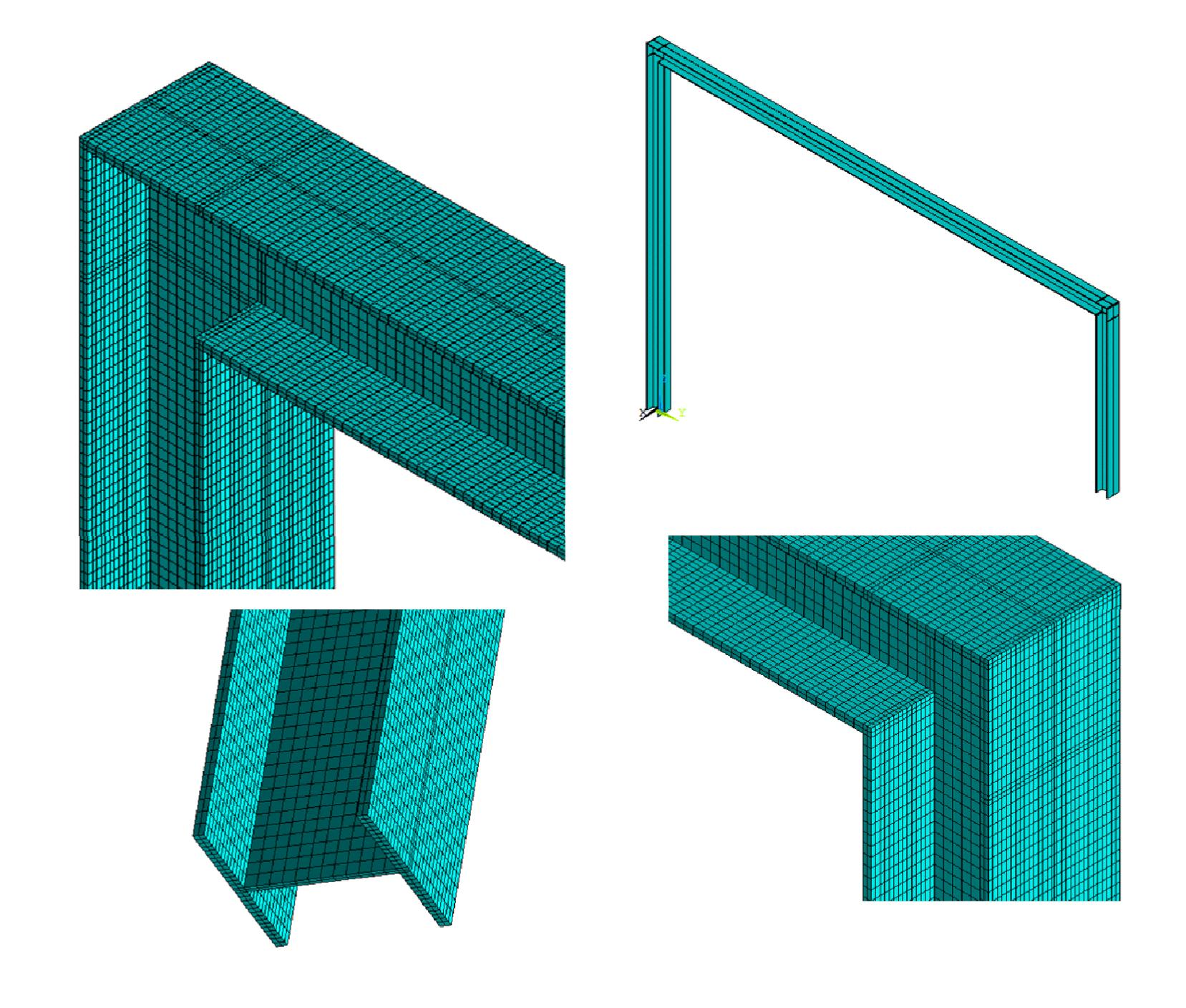Fig.14
Finite element model of the simple portal of Fig. 12: mesh details

In general, numerical investigation of the nonlinear behavior of structures must follow the equilibrium path, identifying and computing the singular points like limit or bifurcation points, whose secondary branches in the equilibrium path must be examined and followed and this procedure can be adversely affected by any kind of approximation, as shown even in the simplest examples (Guarracino, ). To overcome difficulties with limit points, displacement control techniques were introduced and for this reason the modified arc-length method was used in ANSYS to follow the load-deformation path (Forde and Stiemer, ; Crisfield, ).

In fact, the modified arc-length method is suitable for nonlinear static equilibrium solutions of unstable problems and its applications involve the tracing of a complex path in the load-displacement response into the buckling/post buckling regimes. Mathematically, the modified arc-length method can be viewed as the trace of an equilibrium curve in a space spanned by the nodal displacement variables and the total load factor. During the solution, the modified arc-length method varies the arc-length radius at each arc-length substep according to the degree of nonlinearities that is involved.

ANSYS® calculates the reference arc-length radius from the load increment of the first iteration of the first substep, and if an arc-length solution fails to converge within the prescribed maximum number of iterations, the program automatically bisects and continues the analysis. Bisection continues until a converged solution is obtained or until the minimum arc-length radius is used.

By means of this technique and by assuming the same linear elastic-perfect plastic behavior of the material from the limit analysis, the results shown in Fig. 15 were obtained.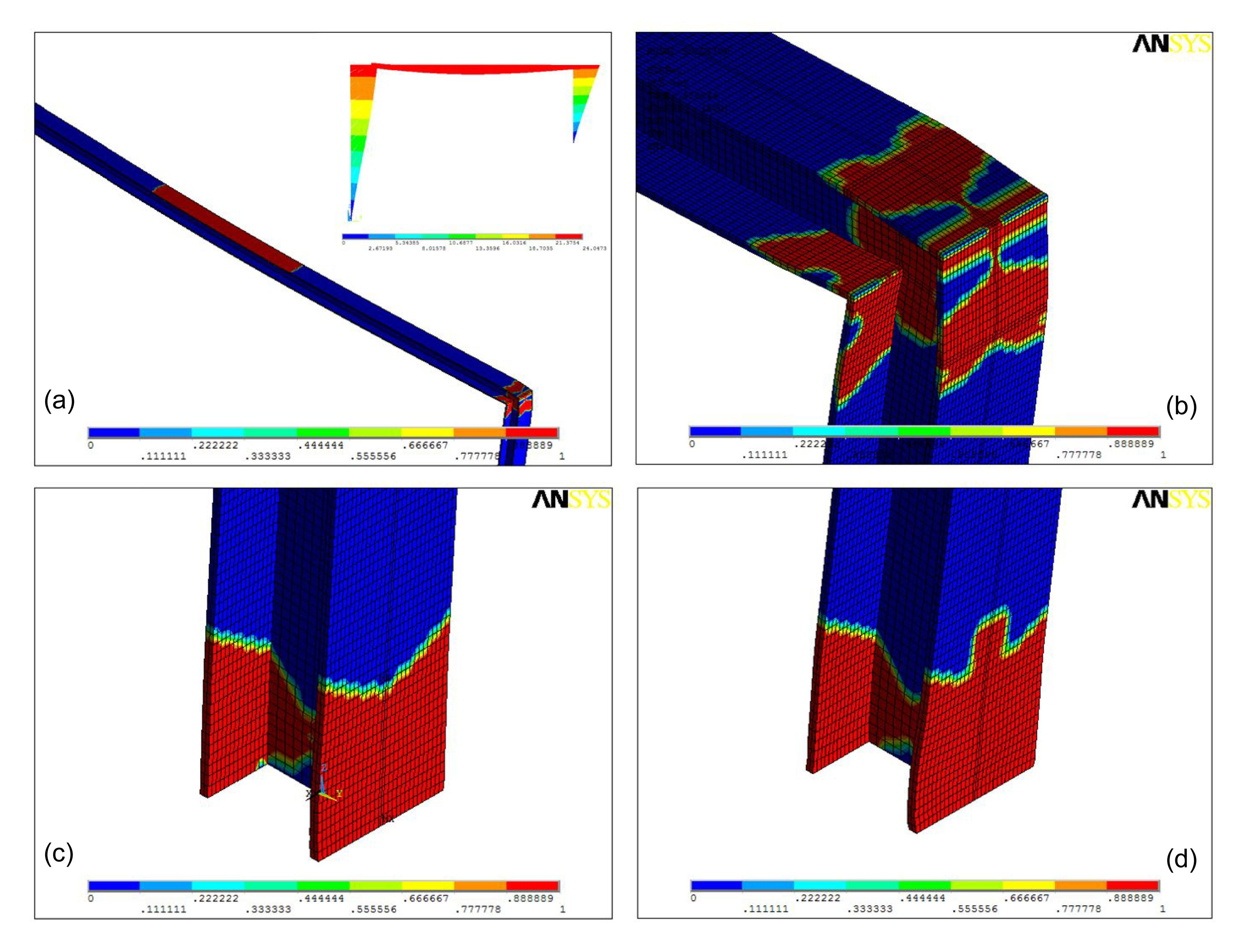Fig.15
Finite element results from nonlinear elastic-plastic analysis with indication of the plastic stress ratio [0-1] (b), (c) and (d). The collapse mechanism (Fig. 15a) is in agreement with that of Fig. 16b from limit analysis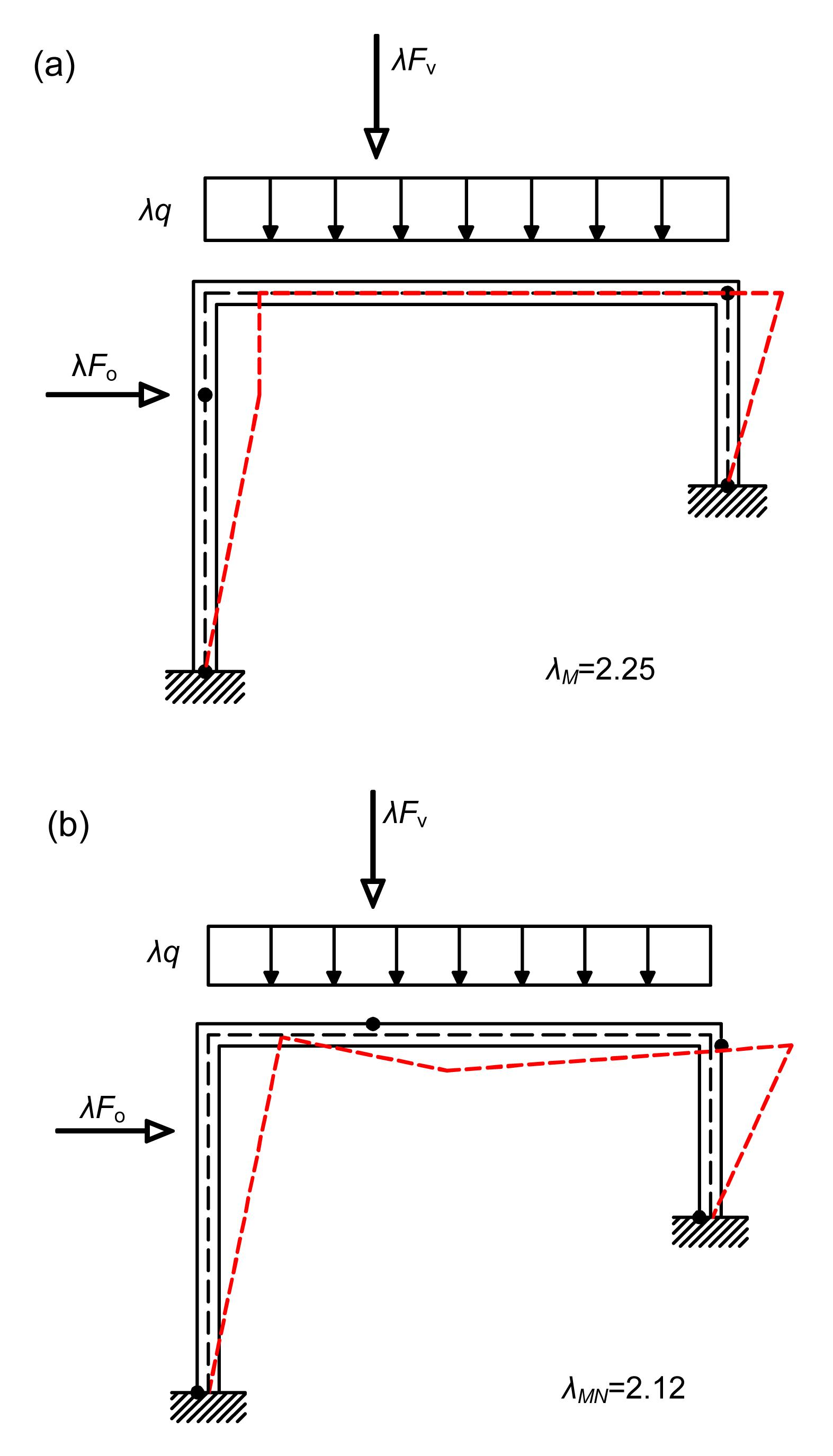Fig.16
Kinematics at collapse for pure bending (a) and for bending moment and axial force interaction (b)

The collapse load multiplier, λ, was found equal to about 1.85 and the localization of the plastic regions at some critical sections clearly suggests the initiation of a mechanism which can be thought as activated by plastic hinges (Fig. 15). The collapse mechanism (Fig. 15a) is in very good agreement with that of Fig. 16b from limit analysis.

Fig. 16 shows the results for the same problem of Fig. 12 from the methods of limit analysis. It is worth noting that, as it was easily expected, both the results from the limit analysis overestimate the load collapse factor. This can be attributed to different factors which are taken into account in the more refined FE analysis, such as the role of geometric nonlinearities, a different stress distribution, and local instabilities of the I beam flanges, as visible in Fig. 15c.

One could therefore wonder about the actual dependability of a limit analysis which, by its own nature, deals, as illustrated, with an extremely simplified model of the structure. However, without indulging in considerations which have been conveyed in a number of studies during the last decades, for the purposes of the present work it can be noted that, on the whole, the kinematics at collapse which can be derived from the FE results of Fig. 15 is essentially the same as Fig. 16b. This suffices to suggest that, albeit the strong idealization at the basis of any limit analysis approach, the key point in replicating what can be thought as the actual collapse mechanisms lies in properly accounting for the axial force-bending moment interaction at the plastic hinges.

This is also confirmed with references to Figs. 17 and 18. Fig. 17 shows the stress distribution in the section at the bottom of the right pillar from the FE analysis, while Fig. 18 shows the same stress distribution from the limit analysis.Fig.17
Results from nonlinear FE analysis at section A: plot of axial stress at impending collapse (unit: daN/cm2)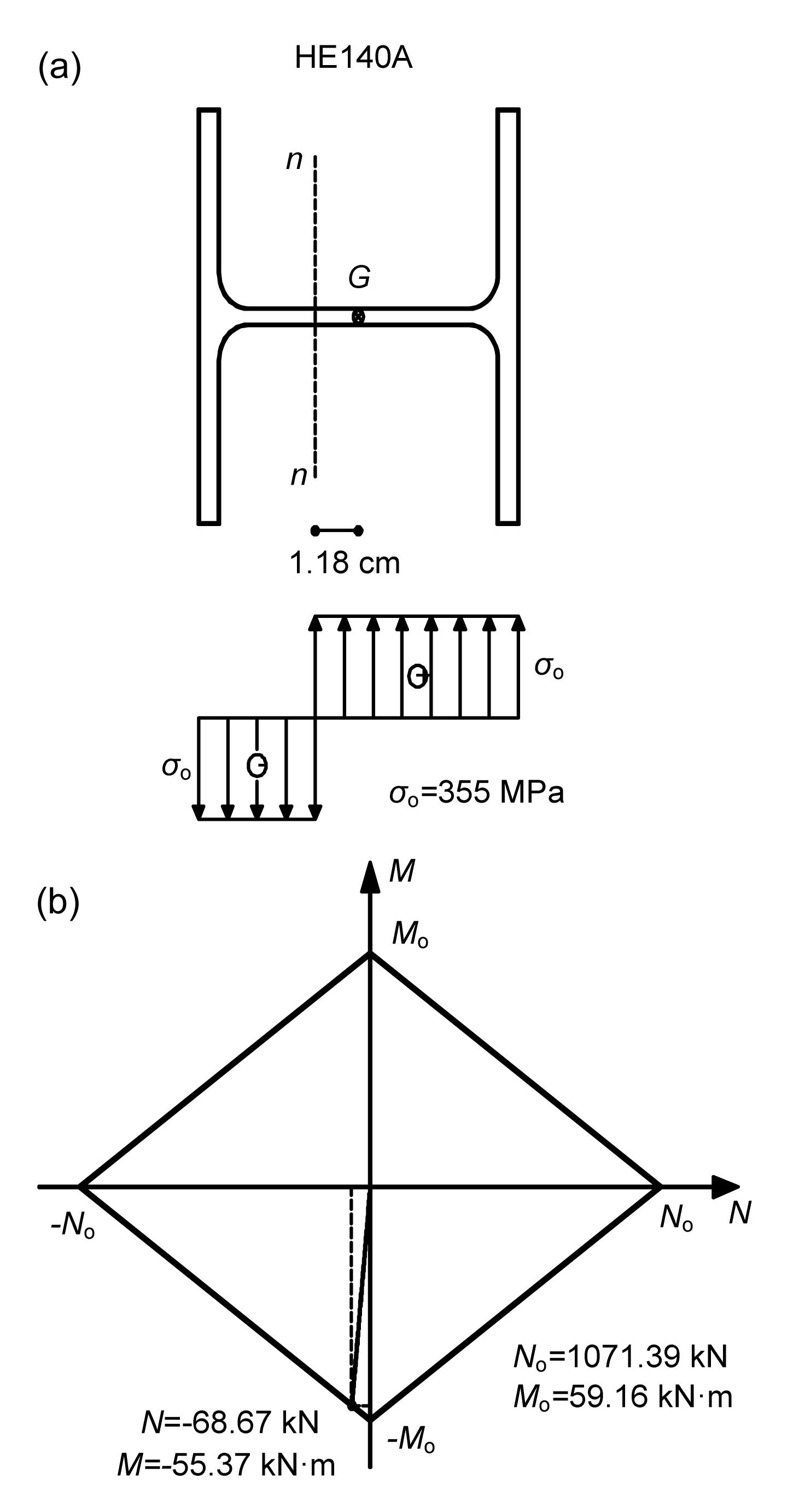Fig.18
Results from limit analysis at section A: axial stress at impending collapse (a) and M-N vector in the limit domain (b)

5.  A simple limit analysis procedure by means of MATHEMATICA®

On the basis of the previous observations, a simple procedure for the limit analysis of ductile plane frames has been written, which accounts in a straight and direct manner for the kinematics stemming from the axial force-bending moment interaction at the plastic hinges.

From the authors’ standpoint, its merit resides in being a simple and efficient tool to assess the ultimate loading capacity which can help to figure out the actual kinematics at collapse as a result of the interaction of the bending moment and axial force in a very straightforward manner and within the framework of the current European codes.

Following what has been previously illustrated, the condition of admissibility at the beam cross sections is taken in the form of Eq. (1) , so that the deformation at the fully plastic sections can be written according to the normality rule , where , where is the plastic rate deformation magnitude, is the vector representing the plastic deformation at the section Si, and is the corresponding normal to the yield surface .

Once an appropriate number of critical sections have been chosen, an equilibrated status of generalised stress can be written as where the vectors Mcc and Ncc collect the values of the bending moments and of the axial forces at the critical sections, f collects the applied loads, and is the load multiplier. x collects the redundancies, i.e., the indeterminacy parameters, and are the basic and redundant load matrices, respectively, for bending, and finally and are the basic and redundant load matrices, respectively, for the axial stress.

The optimization problem simply consists, as known, in maximizing the load multiplier λ within the constraints represented by Eq. (8) at all critical sections (Cohn and Maier, ).

The implementation of this limit analysis procedure has been made with the aid of a symbolic package, MATHEMATICA®, as shown in Fig. 19, where

1. Mcck (Ncck) are the n-dimensional array of bending moments (axial forces) at the chosen control sections in equilibrium with the external actions for each different load case (LC);

2. MFcck (NFcck) are the n-dimensional array of bending moments (axial forces) at the chosen control sections for each different load case (LC) in the frame made statically determined;

3. is the n-dimensional array of self-equilibrated bending moment (axial force) at the chosen control sections in the frame made statically determined , n SE is the number of control sections;

4. Xj are multipliers of the self-equilibrated stress distributions ;

5. AccMk or AccNk are the relationships which represent the yield loci for the control sections in case of bending only (M) or axial force-bending interaction (M-N);

6. SoluzOptccMk or SoluzOptccM Nk is the solution to the constrained linear optimization problem under bending only (M) or axial force-bending interaction (M-N), which makes the load multiplier, λ, a maximum.Fig.19
Implementation of core limit analysis procedure by MATHEMATICA®

It is clear that, according to the procedure of Fig. 19, the rotations at the plastic hinges take place as expected about the pins at the neutral axis of the fully plastic cross sections.

6.  Analysis of a multi-story plane frame

The procedure presented in Section 5 has been used to analyze the plane frame of Fig. 20, subject to vertical and horizontal loads. The limit load multiplier, λ, and the displacement at collapse are shown in Figs. 2124 (p.493) for a pure bending (λM ) mechanism and a bending moment and axial force interaction (λMN ) mechanism for different span lengths. Red dots show plastic hinges in columns, and green dots show plastic hinges in beams.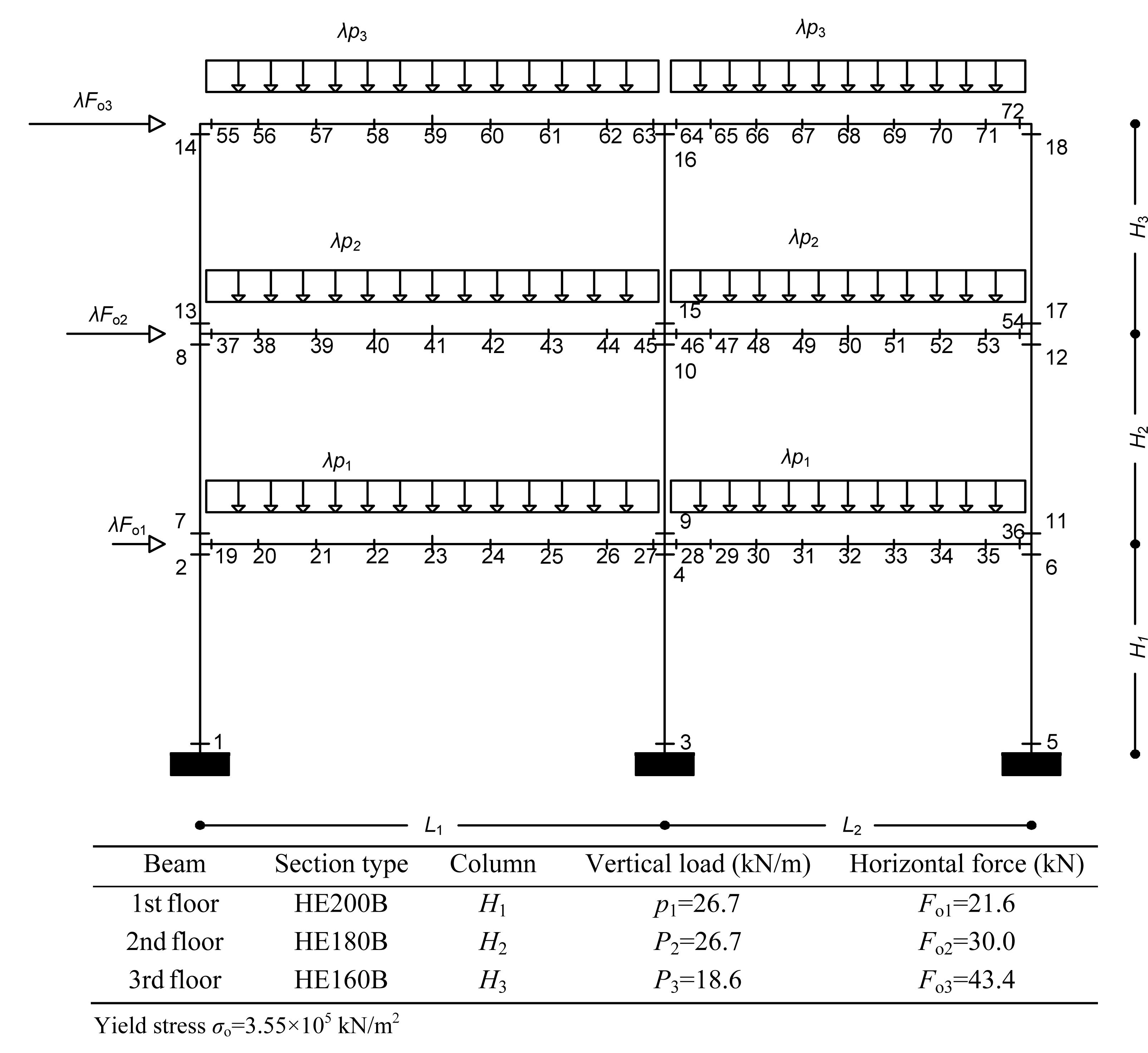Fig.20
A plane frame under vertical and horizontal loads

It is evident that the collapse kinematics can vary significantly and the difference between the respective collapse load multipliers, λM and λMN , ranges from 1.97% to 19.05%.

In the case of Fig. 21, it is L 1=L 2=5 m and the collapse mechanism arising from the plastic hinges located at the center of the mass of the cross sections involving the top three stories, while the mechanism arising from the plastic hinges located at the neutral axis of the cross sections involves the whole frame and is therefore a global one. This fact gives origin to a 19.05% difference between the corresponding values of the load multipliers.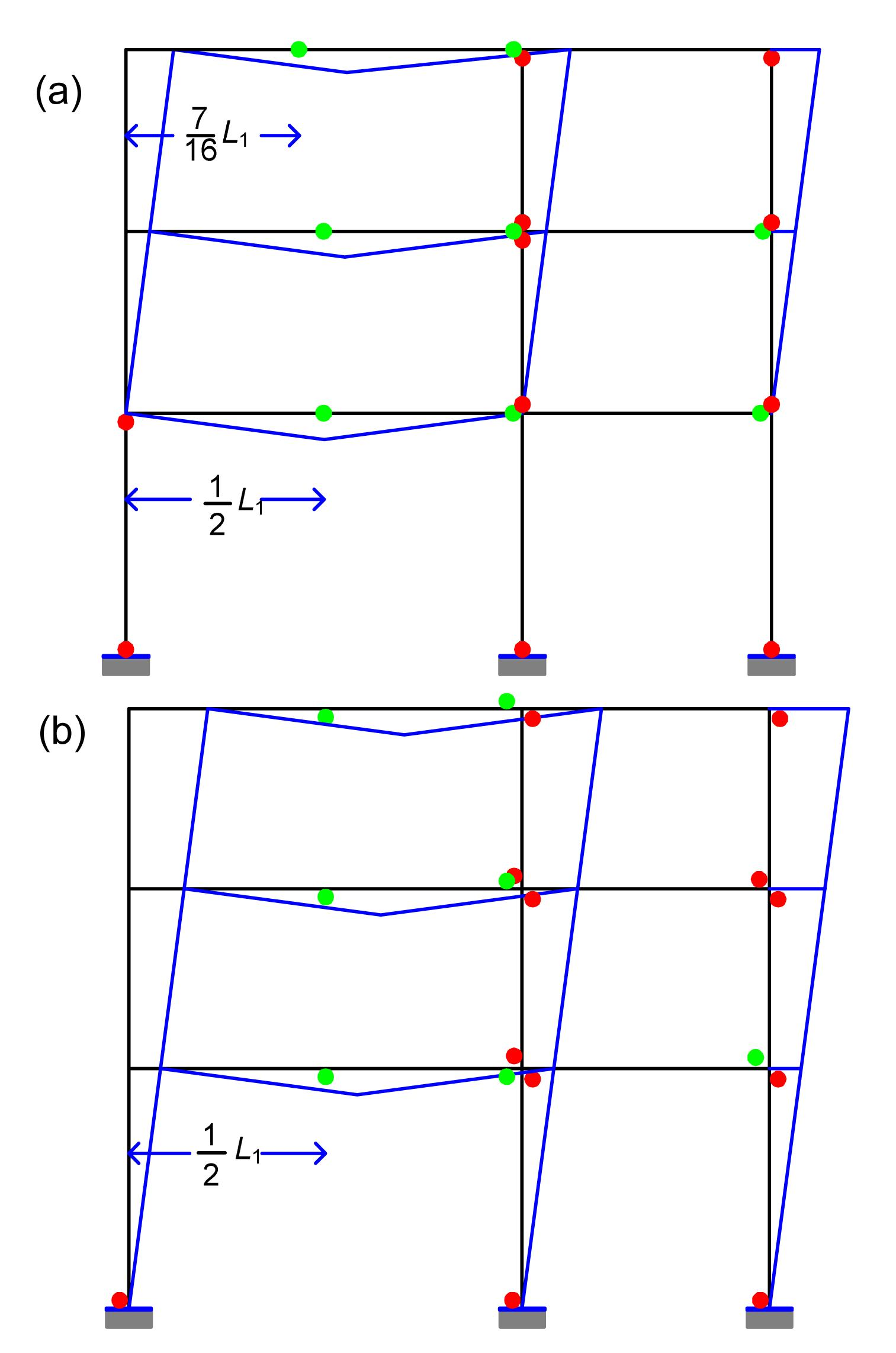Fig.21
Load multiplier and kinematics at collapse for pure bending (λM ) (a) and for bending moment and axial force interaction (λMN ) (b)
L 1=5 m, L 2=5 m, λM =4.66, λMN =3.78, and Δλ=19.05%

In the case of Fig. 22, it is L 1=7 m and L 2=5 m and both collapse mechanisms involve the whole frame with a difference between the corresponding values of the load multipliers which reduces to a mere 1.97%.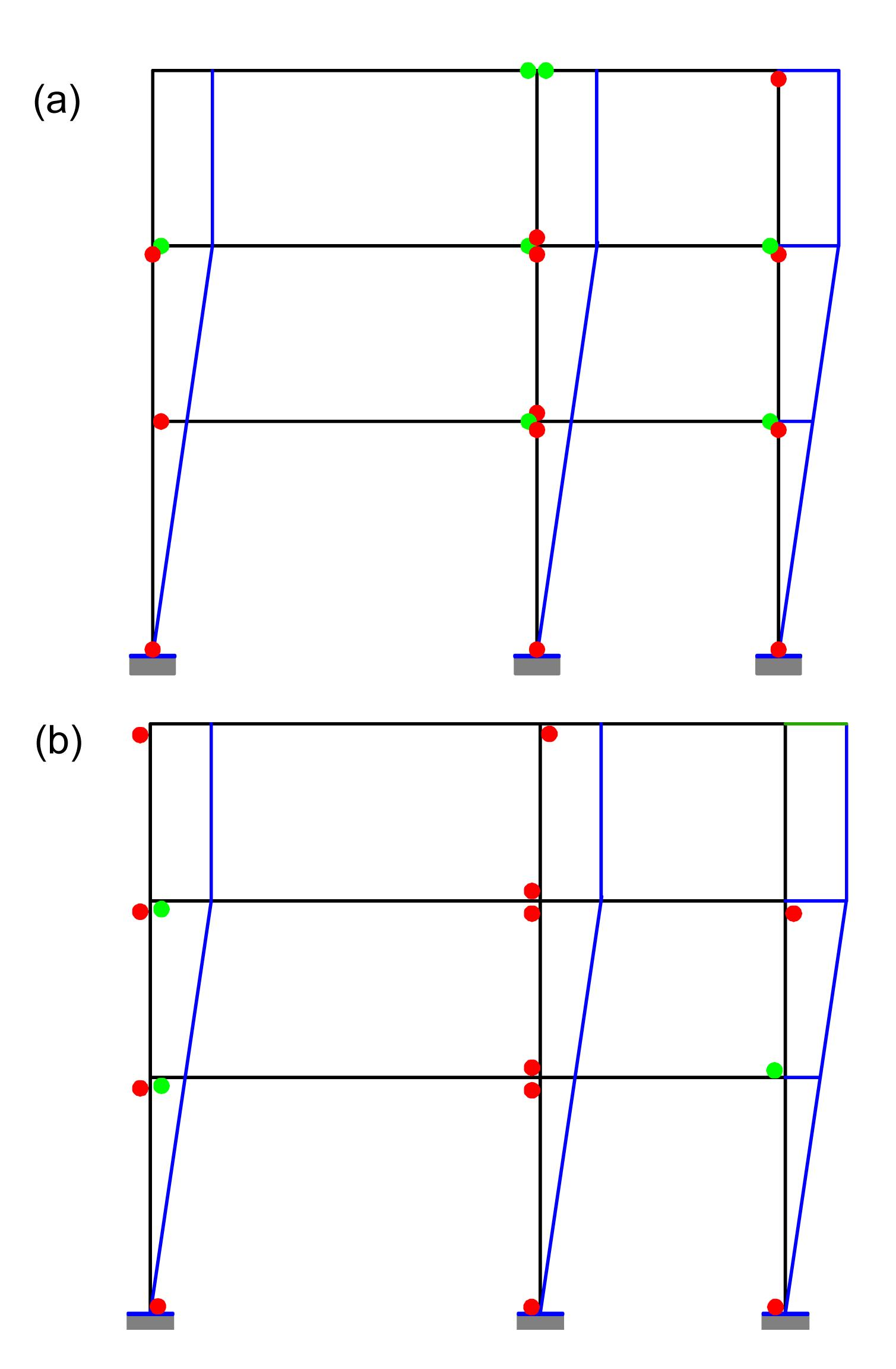Fig.22
Load multiplier and kinematics at collapse for pure bending (λM ) (a) and for bending moment and axial force interaction (λMN ) (b)
L 1=7 m, L 2=5 m, λM =4.55, λMN =4.46, and Δλ=1.97%

In the case of Fig. 23, it is L 1=8 m and L 2=5 m and the collapse mechanisms are again different: the one arising from the plastic hinges located at the center of mass of the cross sections involves the top three stories and the one arising from the plastic hinges located at the neutral axis of the cross sections is again a global mechanism. In this case the difference between the corresponding values of the load multipliers is 8.08%.Fig.23
Load multiplier and kinematics at collapse for pure bending (λM ) (a) and for bending moment and axial force interaction (λMN ) (b)
L 1=8 m, L 2=5 m, λM =2.55, λMN =2.35, and Δλ=8.08%

In the case of Fig. 24, it is L 1=10 m and L 2=8 m and the collapse arising from the plastic hinges located at the center of the mass of the cross section is localized at an horizontal beam, while the one arising from the plastic hinges located at the neutral axis of the cross sections is once again a global mechanism. However, in this case the difference between the corresponding values of the load multipliers is 5.89%.Fig.24
Load multiplier and kinematics at collapse for pure bending (λM ) (a) and for bending moment and axial force interaction (λMN ) (b)
L 1=10 m, L 2=8 m, λM =1.67, λMN =1.57, and Δλ=5.89%

From the few cases shown in Figs. 2124, it is evident that inaccurately accounting for the location of the plastic hinges through the cross section can point to different kinematics at collapse, and therefore great care must be adopted in foreseeing the actual collapse mechanisms. In fact, an incorrectly calculated collapse mechanism may lead to an improper design of new structures or to an inadequate strengthening of existing ones.

7.  Conclusions

The sensitivity of the limit load and of the collapsing modes of the ductile frames to the actual kinematics arising from plastic hinges on account of the axial force-bending moment interaction in presence of combined vertical and horizontal forces, has been pointed out by means of two elementary examples and successively assessed by means of a nonlinear FE analysis and of a straightforward implementation of limit analysis procedures, with the aid of the symbolic code MATHEMATICA®.

It has been shown that even in the simplest cases, the main outcome from limit analysis should consider the kinematics at collapse, which is relevant for both design and reinforcement purposes, within the framework of some standardization codes. If proper accounting is not made for the axial force-bending moment interaction for all the elements of the structure, the calculated localization of the plastic hinges may give origin to incorrect collapse mechanisms and to misleading safety factors. Though focusing on a specific aspect, the study clearly shows that great care must be adopted in the design of new structures or in the strengthening of existing ones, even when using seemingly well-established classical procedures. The proposed approach may be relevant to practising engineers dealing with code prescriptions and standardization committees.

ReferencesAbbas, H., Jones, N., 2012. Influence of strain hardening on bending moment-axial force interaction. International Journal of Mechanical Sciences, 55(1):65-77.ANSYS, 2011.  ANSYS 13.0 Users Documentation. Canonsburg, PA 15317,USA :Baptista, A.M., 2012. Resistance of steel I-sections under axial force and biaxial bending. Journal of Constructional Steel Research, 72:1-11.Baptista, A.M., Muzeau, J.P., 2006. Analytical formulation of the elastic-plastic behaviour of bisymmetrical steel shapes. Journal of Constructional Steel Research, 62(9):872-884.Baptista, A.M., Muzeau, J.P., 2008. Analytical formulation for the deformations of I-shapes and RHS at the plastic strain ultimate limit state. Journal of Constructional Steel Research, 64(10):1165-1177.Chen, W.F., 1988.  Plasticity for Structural Engineers. Springer,New York :Cohn, M.Z., Maier, G., 1979. Engineering Plasticity by Mathematical Programming. , Proceedings of the NATO Advanced Study Institute, University of Waterloo, Canada, :Crisfield, M.A., 1997. Non-linear Finite Element Analysis of Solids and Structures.  Advanced Topics. John Wiley & Sons,Chichester 2:Davies, J.M., 2002. Second-order elastic-plastic analysis of plane frames. Journal of Constructional Steel Research, 58(10):1315-1330.Davies, J.M., 2006. Strain hardening, local buckling and lateral-torsional buckling in plastic hinges. Journal of Constructional Steel Research, 62(1-2):27-34.EC3, 2005.  EN 1993, Eurocode 3: Design of steel structures, Part 1.1: General rules and rules for buildings. European Committee for Standardization (CEN),Brussels :Forde, W.R.B., Stiemer, S.F., 1987. Improved arc length orthogonality methods for nonlinear finite element analysis. Computers & Structures, 27(5):625-630.Fraldi, M., Nunziante, L., Gesualdo, A., 2010. On the bounding of limit multipliers for combined loading. Proceedings of the Royal Society A: Mathematical, Physical and Engineering Sciences, 466:493-514.Gardner, L., Nethercot, D.A., 2005.  Designers guide to EN1993-1-1: Eurocode 3: design of steel structures. Thomas Telford,London :Guarracino, F., 2007. Considerations on the numerical analysis of initial post-buckling behaviour in plates and beams. Thin-walled Structures, 45(10-11):845-848.Guarracino, F., Walker, A.C., 2008. Some comments on the numerical analysis of plates and thin-walled structures. Thin-walled Structures, 46(7-9):975-980.Guarracino, F., Minutolo, V., Nunziante, L., 1992. A simple analysis of soil-structure interaction by BEM-FEM coupling. Engineering Analysis with Boundary Elements, 10(4):283-289.Halleux, J.P., 1981.  EUR 7329 EN. Nuclear science and technology. Modelling of elasto-plastic material behaviour. Commission of the European Communities,Joint Research Centre, Ispra, Italy :Kim, K.P., Huh, H., 2006. Dynamic limit analysis formulation for impact simulation of structural members. International Journal of Solids and Structures, 43(21):6488-6501.Kwasniewski, L., 2010. Nonlinear dynamic simulations of progressive collapse for a multistory building. Engineering Structures, 32(5):1223-1235.Massonnet, C., Save, M., 1976. Calcul Plastique des Constructions. Vol. 1: Structures Dpendant d'un Paramtre, (in French), Nelissen, Lige :Neal, B.G., 1963.  The Plastic Methods of Structural Analysis. Chapman and Hall,London :Petrolito, J., Legge, K., 2012. Benchmarks for elastoplastic analysis of steel frames. Australian Journal of Structural Engineering, 12(3):237-250.Save, M., de Saxce, G., Borkowski, A., 1991. EUR 13618 EN. Nuclear science and technology. Computation of shakedown loads feasibility study, Final Report, Commission of the European Communities,:Stssi, F., Kollbrunner, C.F., 1935. Beitrag zum traglastverfahren. Die Bautechnik, (in German),13(21):264-267.Trahair, N.S., Bradford, M.A., Nethercot, D.A., 2008. The Behaviour and Design of Steel Structures to EC3, CRC Press,:Wolfram, S., 2003.  The Mathematica Book. Wolfram Media,Champaign, IL :

<1>

2014-07-09 14:58:54

it's perfect